# Site Swap

There is a mathematical notation for juggling that describes the possible juggling patterns for a given number of balls. The notation is called site swap notation, alternatively written as a single word siteswap. The numbers are an abstract short hand. The numbers do not tell you how to juggle, the numbers do not tell you where to move your hands, nor whether to throw balls over or under other balls.

A simple three ball cascade juggle has each of the three balls passing through each of the hands in alternation. Each hand must touch each ball in succession, with the balls being passed from the right to the left and back to the right. The first ball thrown must "fill" the right hand after the third ball has left the right hand.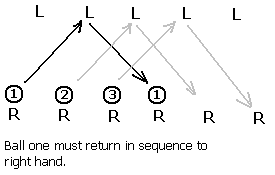In the above space-time diagram time is passing as one moves from left to right.

If we "interleave" the left hands inbetween the right hands thusly: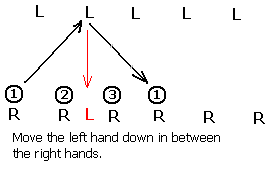...we obtain the following diagram: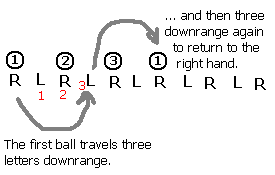Studying the above diagram we can see that the ball moves three "hands" downrange before landing on the other hand (think of hands as landing sites, hence the use of the word site in the name of the notation). The first ball returns to the right hand after moving three more hands (or sites) rightward in the diagram.

There are three basic patterns in juggling.

• Cascade: each hand throws in alternation to the other hand in a left-right pattern. In the simplest form, balls all rise to the same height.
• Shower: one hand throws the balls in an arc typically higher than a cascade, while the other hands always catches the balls and passes them back to the first hand. The pass back is barely a throw.
• Columns: the objects are juggled in vertical columns. For three balls, this means that one ball moves between the left and right hand while the other two hop up and down staying always on the left or right.
• Fountain: Done with four or more even numbers of balls, the balls always thrown and caught by the same hand.

In the cascade each ball follows this exact same symmetrical pattern. Mathematically this is recorded as 3 3 3 3 3 3 3 3 3... To save space, we toss the spaces and write 33333... For further simplicity we invoke a rule that number patterns repeat indefinitely, hence a single 3 can be used to denote this pattern.

Another pattern is the three ball shower, with the balls always being thrown from one hand and then caught and fed back to the first hand in a transfer. The right hand must "launch" the balls in sequence "downrange" to the opposite hand. The first opposite open "site" (hand) that permits a transfer (a throw of 1) to set up the relaunch of ball one is the fifth site.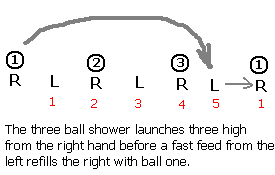Mapping the launches and landings of the other two balls confirms that the balls land "5 site" downrange. This is refered to as a 5 1, or 51 with the spaces removed, with each ball executing the same 51 pattern over and over: 515151...

Other notes:

• Throws alternate hands. The basic site swap system does not take into account synchronous throws.
• A zero is an empty hand.
• Even numbers return to the same hand. A 2 is held in the hand for a "beat", it is not a thrown ball. Think of it as a pause.
• There are n-1 catch/tosses prior to a ball landing.
• Site swap numbers can be conceived of as "beats."
• For the number 3 and above, the height of the throw is related to the number. The relationship is NON-LINEAR.

### The swapping

The notation is called site swap in part because one can "swap" or "switch around" the "sites" (hands!) on which balls land. That it, from a three ball cascade one can toss a ball from the right hand straight up, catching the ball four sites (or four counts or four beats) later. This gives one time to catch and throw a ball from the left hand while the 4 count ball is airborne. While this is happening the left hand holds onto a ball. This is counted as a "2" in the world site swaps (even numbers return to the same hand, holding a ball for an extra beat results in calling this a count of 2).

The diagram depicts a start of a three ball cascade with a single column ball off the right hand followed by a return to a three ball cascade. The notation, unsimplified, would be 33423333...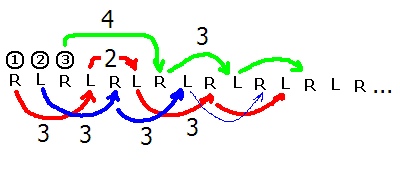## The math

• For any site swap number sequence the average must be equal to the number of balls.
• Two balls must not come down into the same hand at the same time. This rules out sequences such as a 3 2 1 (321). This rule is far harder to check and generally requires making a space-time diagram or analyzing the orbits of individual balls.

To determine the number of balls calculate the average for each sequence. Add the numbers as single digits and then divide by the number of numbers. [Note to real site swappers: this is intended as math exercise and the author does not mean to imply that this condition is by any means sufficient. It is not sufficient. The class will not be checking for multiplex landings, transition issues, or excited states.]

Determine the number of balls in the following patterns. If the mean is fractional, then the pattern does not exist or is incomplete.

1. 3 Pattern exists? _______ Number of balls: _________
2. 51 Pattern exists? _______ Number of balls: _________
3. 342 Pattern exists? _______ Number of balls: _________
4. 552 Pattern exists? _______ Number of balls: _________
5. 333342333 Pattern exists? _______ Number of balls: _________
6. 20 Pattern exists? _______ Number of balls: _________
7. 31 Pattern exists? _______ Number of balls: _________
8. 32 Pattern exists? _______ Number of balls: _________
9. 52 Pattern exists? _______ Number of balls: _________
10. 771 Pattern exists? _______ Number of balls: _________
11. 766 Pattern exists? _______ Number of balls: _________
12. 5 Pattern exists? _______ Number of balls: _________
13. 501 Pattern exists? _______ Number of balls: _________
14. 322 Pattern exists? _______ Number of balls: _________
15. 744 Pattern exists? _______ Number of balls: _________
16. 441 Pattern exists? _______ Number of balls: _________

### Joggling

Running and juggling is difficult with anything other than patterns that synchronize with the cadence of the runner. Assymetrical patterns such as 51 are very difficult to maintain while running. Since cadence is essentially a two beat, left right proposition, this author suspects that only patterns of single digits N M where N=M are really functional. This is potentially a massive limitation leaving only simple cascades as a functional possibility.